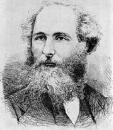Home

Lecture Notes

PHY 218: Electricity and Magnetism II
Prof. S. Teitel stte@pas.rochester.edu ---- Spring 2017

## Lecture Notes

The lecture note files correspond roughly to the material presented in a given day's lecture. But you may on occassion find the end of one day's lecture at the start of the file for the next day's lecture, so please look there if you think there might be something missing.

• Lecture 0 - A brief history of electromagnetism

• Lecture 1 - Review of electro- and magneto- statics, electromotive force, electromagnetic induction in a loop moving in a static magnetic field, Faraday's Law, Lenz' Law

• Lecture 2 - Magnetic flux, E from a ∂B/∂t, Levi-Civita symbol, mutual and self inductance, LR circuit

• Lecture 3 - Energy stored in a magnetostatic current configuration and the force between two current carrying loops

• Lecture 4 - Examples

• Lecture 5 - Maxwell's correction to Ampere's Law, conservation of energy in presence of electromagnetic fields, electromagnetic energy density and electromagnetic energy current (Poynting vector)

• Lecture 6 - Conservation of momentum, Maxwell stress tensor, electromagnetic momentum density and angular momentum density, magnetic monopoles

• Lecture 7 - Maxwell's equations in potential form, gauge transformations, Coulomb gauge, Lorentz gauge, electromagnetic waves in a vacuum

• Lecture 8 - Solutions to the wave equation, plane waves, spherical waves, simple harmonic wave, Fourier transform, general solution to the homogeneous wave equation

• Lecture 9 - Green's function for the inhomogeneous wave equation, longitudinal, transverse, and circular polarization, electromagnetic waves in a vacuum, energy and momentum of EM waves in a vacuum, bound current from time varying polarization density

• Lecture 10 - Macroscopic Maxwell's equations in matter, wave in a linear material with constant permeability and permeativity, frequency dependent polarizability, electric susceptibility and permittivity, non-local in time relation between displacement field D and electric field E

• Lecture 11 - Waves in a dielectric, dispersion relation, effects of complex permittivity, phase velocity, group velocity, normal and anomalous dispersion, wave pulse spreading

• Lecture 12 - Real and imaginary parts of the permittivity, real and imaginary parts of the wavenumber, regions of transparent propagation, resonant absorption, and total reflection

• Lecture 13 - Waves in a conductor, free current density and free charge density, frequency dependent conductivity, dispersion relation, good and poor conductors, skin depth, plasma frequency

• Lecture 14 - Longitudinal modes in a conductor, plasma oscillations, reflection and transmission of waves at an interface, Snell's law, critical angle, total internal reflection

• Lecture 15 - Corrections to Snell's law for a dissipative medium, transmission into a highly absorptive medium, reflected and transmitted field amplitudes, coefficient of reflection, region of total reflection

• Lecture 16 - Reflection between two transparent media, Brewster's angle, Green's function for the wave equation

• Lecture 17 - Radiation from a localized oscillating charge density, expansion for the vector potential, electric dipole, magnetic dipole and electric quadrapole terms for radiation

• Lecture 18 - Relative sizes of dipole and quadrapole terms, electric and magnetic fields in the electric dipole approximation, radiation zone limit, Poynting vector and radiated power in the electric dipole approximation, why is the sky blue?

• Lecture 19 - Magnetic dipole radiation, radiation from an arbitrary time-dependent charge distribution, Larmor's formula for the radiated power of an accelerated charge
Supplement - Computing the Greens function for the wave equation in real space-time

• Lecture 20 - Radiation-reaction force, radiative decay of a classical atom, Lienard-Wiechert potentials for a moving point charge
Supplement - The Abraham-Lorentz Equation - we did not cover this in lecture, and you're not responsible for it, but it is interesting so here it is!

• Lecture 21 - Potentials and fields for a point charge moving with constant velocity, and for a charge accelerating

• Lecture 22 - Special relativity, Lorentz transformation, time dilation, FitzGerald contraction, simultaneity of events, proper time, proper length

• Lecture 23 - 4-vectors, Lorentz transformation matrix, 4-differential, proper time interval, 4-velocity, 4-acceleration, 4-gradient, wave equation operator, 4-current, 4-potential

• Lecture 24 - The field strength tensor Fμν, transformation law for E and B fields, Maxwell's equations in relativistic form, energy-momentum 4-vector, Minkowski force, relativisitc kinetic energy

• Lecture 25 - Conservation of energy and momentum, the Lorentz force in relativistic form, the relativisitic generalization of Larmor's formula

• Lecture 26 - Lienard-Wiechert potentials in relativistic form, field strength tensor and E and B fields for a charged particle moving on an abritrary trajectory

• Lecture 27 - Angular distribution of radiated power from an accelerating charge, non-relativistic limit, linear motion, circular motion